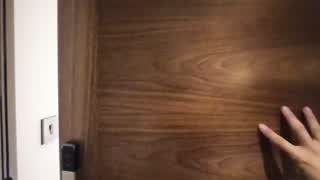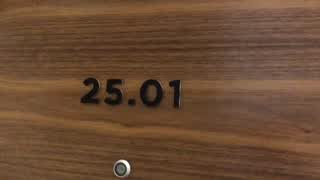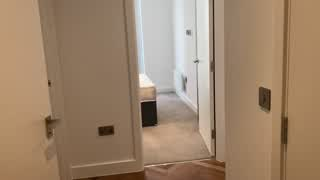# HL-South Tower微信扫一扫，小程序查看公寓£349 /周

37 位学生已收藏此公寓

• 地址:

Owen St, Manchester, 曼彻斯特, M15 4RT

• 前往英国及爱尔兰现代音乐学院（曼彻斯特校区）：
• 13分钟
• 10分钟
• 4分钟
• 前往曼彻斯特都会大学：
• 15分钟
• 15分钟
• 4分钟
• 设施: 提供家具, 洗衣房, 冰箱, 健身房
• 房租包含: 无线网, 水费, 电费, 暖气, 煤气费
• ## 设施&服务

设施
• 无线网络
• 电影放映室
• 健身房
• 冰箱
• 自行车车库
• 前台
• 公寓经理
• 电梯
• 娱乐区/室
• 提供家具
• 套房内洗衣设施
安全&保障
• 闭路电视/监控摄像机
• 安全警报
• 维修团队（24小时待命）
• 安保人员（24小时巡逻）
• 安全门禁（电子钥匙）
公寓规定
• 禁止吸烟
• 禁止饲养宠物
公寓规定
• 禁止吸烟
• 禁止饲养宠物
公寓规定
• 禁止吸烟
• 禁止饲养宠物
公寓规定
• 禁止吸烟
• 禁止饲养宠物
公寓规定
• 禁止吸烟
• 禁止饲养宠物
公寓规定
• 禁止吸烟
• 禁止饲养宠物
公寓规定
• 禁止吸烟
• 禁止饲养宠物
公寓规定
• 禁止吸烟
• 禁止饲养宠物
公寓规定
• 禁止吸烟
• 禁止饲养宠物
公寓规定
• 禁止吸烟
• 禁止饲养宠物
公寓规定
• 禁止吸烟
• 禁止饲养宠物
公寓规定
• 禁止吸烟
• 禁止饲养宠物
公寓规定
• 禁止吸烟
• 禁止饲养宠物
公寓规定
• 禁止吸烟
• 禁止饲养宠物
公寓规定
• 禁止吸烟
• 禁止饲养宠物
公寓规定
• 禁止吸烟
• 禁止饲养宠物
公寓规定
• 禁止吸烟
• 禁止饲养宠物
公寓规定
• 禁止吸烟
• 禁止饲养宠物
公寓规定
• 禁止吸烟
• 禁止饲养宠物
公寓规定
• 禁止吸烟
• 禁止饲养宠物
公寓规定
• 禁止吸烟
• 禁止饲养宠物
公寓规定
• 禁止吸烟
• 禁止饲养宠物
公寓规定
• 禁止吸烟
• 禁止饲养宠物
公寓规定
• 禁止吸烟
• 禁止饲养宠物
公寓规定
• 禁止吸烟
• 禁止饲养宠物
公寓规定
• 禁止吸烟
• 禁止饲养宠物
公寓规定
• 禁止吸烟
• 禁止饲养宠物
公寓规定
• 禁止吸烟
• 禁止饲养宠物
公寓规定
• 禁止吸烟
• 禁止饲养宠物
公寓规定
• 禁止吸烟
• 禁止饲养宠物
公寓规定
• 禁止吸烟
• 禁止饲养宠物
公寓规定
• 禁止吸烟
• 禁止饲养宠物
公寓规定
• 禁止吸烟
• 禁止饲养宠物
公寓规定
• 禁止吸烟
• 禁止饲养宠物
公寓规定
• 禁止吸烟
• 禁止饲养宠物
公寓规定
• 禁止吸烟
• 禁止饲养宠物
公寓规定
• 禁止吸烟
• 禁止饲养宠物
公寓规定
• 禁止吸烟
• 禁止饲养宠物
公寓规定
• 禁止吸烟
• 禁止饲养宠物
公寓规定
• 禁止吸烟
• 禁止饲养宠物
公寓规定
• 禁止吸烟
• 禁止饲养宠物
公寓规定
• 禁止吸烟
• 禁止饲养宠物
公寓规定
• 禁止吸烟
• 禁止饲养宠物
公寓规定
• 禁止吸烟
• 禁止饲养宠物
公寓规定
• 禁止吸烟
• 禁止饲养宠物
公寓规定
• 禁止吸烟
• 禁止饲养宠物
公寓规定
• 禁止吸烟
• 禁止饲养宠物
公寓规定
• 禁止吸烟
• 禁止饲养宠物
公寓规定
• 禁止吸烟
• 禁止饲养宠物
公寓规定
• 禁止吸烟
• 禁止饲养宠物
公寓规定
• 禁止吸烟
• 禁止饲养宠物
公寓规定
• 禁止吸烟
• 禁止饲养宠物
公寓规定
• 禁止吸烟
• 禁止饲养宠物
公寓规定
• 禁止吸烟
• 禁止饲养宠物
公寓规定
• 禁止吸烟
• 禁止饲养宠物
公寓规定
• 禁止吸烟
• 禁止饲养宠物
公寓规定
• 禁止吸烟
• 禁止饲养宠物
公寓规定
• 禁止吸烟
• 禁止饲养宠物
公寓规定
• 禁止吸烟
• 禁止饲养宠物
公寓规定
• 禁止吸烟
• 禁止饲养宠物
公寓规定
• 禁止吸烟
• 禁止饲养宠物
公寓规定
• 禁止吸烟
• 禁止饲养宠物
公寓规定
• 禁止吸烟
• 禁止饲养宠物
公寓规定
• 禁止吸烟
• 禁止饲养宠物
公寓规定
• 禁止吸烟
• 禁止饲养宠物
公寓规定
• 禁止吸烟
• 禁止饲养宠物
公寓规定
• 禁止吸烟
• 禁止饲养宠物
公寓规定
• 禁止吸烟
• 禁止饲养宠物
公寓规定
• 禁止吸烟
• 禁止饲养宠物
公寓规定
• 禁止吸烟
• 禁止饲养宠物
公寓规定
• 禁止吸烟
• 禁止饲养宠物
公寓规定
• 禁止吸烟
• 禁止饲养宠物
公寓规定
• 禁止吸烟
• 禁止饲养宠物
公寓规定
• 禁止吸烟
• 禁止饲养宠物
公寓规定
• 禁止吸烟
• 禁止饲养宠物
公寓规定
• 禁止吸烟
• 禁止饲养宠物
公寓规定
• 禁止吸烟
• 禁止饲养宠物
公寓规定
• 禁止吸烟
• 禁止饲养宠物
公寓规定
• 禁止吸烟
• 禁止饲养宠物
公寓规定
• 禁止吸烟
• 禁止饲养宠物
公寓规定
• 禁止吸烟
• 禁止饲养宠物
公寓规定
• 禁止吸烟
• 禁止饲养宠物
公寓规定
• 禁止吸烟
• 禁止饲养宠物
公寓规定
• 禁止吸烟
• 禁止饲养宠物
公寓规定
• 禁止吸烟
• 禁止饲养宠物
公寓规定
• 禁止吸烟
• 禁止饲养宠物
公寓规定
• 禁止吸烟
• 禁止饲养宠物
公寓规定
• 禁止吸烟
• 禁止饲养宠物
公寓规定
• 禁止吸烟
• 禁止饲养宠物
公寓规定
• 禁止吸烟
• 禁止饲养宠物
公寓规定
• 禁止吸烟
• 禁止饲养宠物
公寓规定
• 禁止吸烟
• 禁止饲养宠物
公寓规定
• 禁止吸烟
• 禁止饲养宠物
公寓规定
• 禁止吸烟
• 禁止饲养宠物
公寓规定
• 禁止吸烟
• 禁止饲养宠物
公寓规定
• 禁止吸烟
• 禁止饲养宠物
公寓规定
• 禁止吸烟
• 禁止饲养宠物
公寓规定
• 禁止吸烟
• 禁止饲养宠物
公寓规定
• 禁止吸烟
• 禁止饲养宠物
公寓规定
• 禁止吸烟
• 禁止饲养宠物
公寓规定
• 禁止吸烟
• 禁止饲养宠物
公寓规定
• 禁止吸烟
• 禁止饲养宠物
公寓规定
• 禁止吸烟
• 禁止饲养宠物
公寓规定
• 禁止吸烟
• 禁止饲养宠物
公寓规定
• 禁止吸烟
• 禁止饲养宠物
公寓规定
• 禁止吸烟
• 禁止饲养宠物
公寓规定
• 禁止吸烟
• 禁止饲养宠物
公寓规定
• 禁止吸烟
• 禁止饲养宠物
公寓规定
• 禁止吸烟
• 禁止饲养宠物
公寓规定
• 禁止吸烟
• 禁止饲养宠物
公寓规定
• 禁止吸烟
• 禁止饲养宠物
公寓规定
• 禁止吸烟
• 禁止饲养宠物
公寓规定
• 禁止吸烟
• 禁止饲养宠物
公寓规定
• 禁止吸烟
• 禁止饲养宠物
公寓规定
• 禁止吸烟
• 禁止饲养宠物
公寓规定
• 禁止吸烟
• 禁止饲养宠物
公寓规定
• 禁止吸烟
• 禁止饲养宠物
公寓规定
• 禁止吸烟
• 禁止饲养宠物
公寓规定
• 禁止吸烟
• 禁止饲养宠物
公寓规定
• 禁止吸烟
• 禁止饲养宠物
公寓规定
• 禁止吸烟
• 禁止饲养宠物
公寓规定
• 禁止吸烟
• 禁止饲养宠物
公寓规定
• 禁止吸烟
• 禁止饲养宠物
公寓规定
• 禁止吸烟
• 禁止饲养宠物
公寓规定
• 禁止吸烟
• 禁止饲养宠物
公寓规定
• 禁止吸烟
• 禁止饲养宠物
公寓规定
• 禁止吸烟
• 禁止饲养宠物
公寓规定
• 禁止吸烟
• 禁止饲养宠物
公寓规定
• 禁止吸烟
• 禁止饲养宠物
公寓规定
• 禁止吸烟
• 禁止饲养宠物
公寓规定
• 禁止吸烟
• 禁止饲养宠物
公寓规定
• 禁止吸烟
• 禁止饲养宠物
公寓规定
• 禁止吸烟
• 禁止饲养宠物
公寓规定
• 禁止吸烟
• 禁止饲养宠物
公寓规定
• 禁止吸烟
• 禁止饲养宠物
公寓规定
• 禁止吸烟
• 禁止饲养宠物
公寓规定
• 禁止吸烟
• 禁止饲养宠物
公寓规定
• 禁止吸烟
• 禁止饲养宠物
公寓规定
• 禁止吸烟
• 禁止饲养宠物
公寓规定
• 禁止吸烟
• 禁止饲养宠物
公寓规定
• 禁止吸烟
• 禁止饲养宠物
公寓规定
• 禁止吸烟
• 禁止饲养宠物
公寓规定
• 禁止吸烟
• 禁止饲养宠物
公寓规定
• 禁止吸烟
• 禁止饲养宠物
公寓规定
• 禁止吸烟
• 禁止饲养宠物
公寓规定
• 禁止吸烟
• 禁止饲养宠物
公寓规定
• 禁止吸烟
• 禁止饲养宠物
公寓规定
• 禁止吸烟
• 禁止饲养宠物
公寓规定
• 禁止吸烟
• 禁止饲养宠物
公寓规定
• 禁止吸烟
• 禁止饲养宠物
公寓规定
• 禁止吸烟
• 禁止饲养宠物
公寓规定
• 禁止吸烟
• 禁止饲养宠物
公寓规定
• 禁止吸烟
• 禁止饲养宠物
公寓规定
• 禁止吸烟
• 禁止饲养宠物
公寓规定
• 禁止吸烟
• 禁止饲养宠物
公寓规定
• 禁止吸烟
• 禁止饲养宠物
公寓规定
• 禁止吸烟
• 禁止饲养宠物
公寓规定
• 禁止吸烟
• 禁止饲养宠物
公寓规定
• 禁止吸烟
• 禁止饲养宠物
公寓规定
• 禁止吸烟
• 禁止饲养宠物
公寓规定
• 禁止吸烟
• 禁止饲养宠物
公寓规定
• 禁止吸烟
• 禁止饲养宠物
公寓规定
• 禁止吸烟
• 禁止饲养宠物
公寓规定
• 禁止吸烟
• 禁止饲养宠物
公寓规定
• 禁止吸烟
• 禁止饲养宠物
公寓规定
• 禁止吸烟
• 禁止饲养宠物
公寓规定
• 禁止吸烟
• 禁止饲养宠物
公寓规定
• 禁止吸烟
• 禁止饲养宠物
公寓规定
• 禁止吸烟
• 禁止饲养宠物
公寓规定
• 禁止吸烟
• 禁止饲养宠物
公寓规定
• 禁止吸烟
• 禁止饲养宠物
公寓规定
• 禁止吸烟
• 禁止饲养宠物
公寓规定
• 禁止吸烟
• 禁止饲养宠物
公寓规定
• 禁止吸烟
• 禁止饲养宠物
公寓规定
• 禁止吸烟
• 禁止饲养宠物
公寓规定
• 禁止吸烟
• 禁止饲养宠物
公寓规定
• 禁止吸烟
• 禁止饲养宠物
公寓规定
• 禁止吸烟
• 禁止饲养宠物
公寓规定
• 禁止吸烟
• 禁止饲养宠物
公寓规定
• 禁止吸烟
• 禁止饲养宠物
公寓规定
• 禁止吸烟
• 禁止饲养宠物
公寓规定
• 禁止吸烟
• 禁止饲养宠物
公寓规定
• 禁止吸烟
• 禁止饲养宠物
公寓规定
• 禁止吸烟
• 禁止饲养宠物
公寓规定
• 禁止吸烟
• 禁止饲养宠物
公寓规定
• 禁止吸烟
• 禁止饲养宠物
公寓规定
• 禁止吸烟
• 禁止饲养宠物
公寓规定
• 禁止吸烟
• 禁止饲养宠物
公寓规定
• 禁止吸烟
• 禁止饲养宠物
公寓规定
• 禁止吸烟
• 禁止饲养宠物
公寓规定
• 禁止吸烟
• 禁止饲养宠物
公寓规定
• 禁止吸烟
• 禁止饲养宠物
公寓规定
• 禁止吸烟
• 禁止饲养宠物
公寓规定
• 禁止吸烟
• 禁止饲养宠物
公寓规定
• 禁止吸烟
• 禁止饲养宠物
公寓规定
• 禁止吸烟
• 禁止饲养宠物
公寓规定
• 禁止吸烟
• 禁止饲养宠物
公寓规定
• 禁止吸烟
• 禁止饲养宠物
公寓规定
• 禁止吸烟
• 禁止饲养宠物
公寓规定
• 禁止吸烟
• 禁止饲养宠物
公寓规定
• 禁止吸烟
• 禁止饲养宠物
公寓规定
• 禁止吸烟
• 禁止饲养宠物
公寓规定
• 禁止吸烟
• 禁止饲养宠物
公寓规定
• 禁止吸烟
• 禁止饲养宠物
公寓规定
• 禁止吸烟
• 禁止饲养宠物
公寓规定
• 禁止吸烟
• 禁止饲养宠物
公寓规定
• 禁止吸烟
• 禁止饲养宠物
公寓规定
• 禁止吸烟
• 禁止饲养宠物
公寓规定
• 禁止吸烟
• 禁止饲养宠物
公寓规定
• 禁止吸烟
• 禁止饲养宠物
公寓规定
• 禁止吸烟
• 禁止饲养宠物
公寓规定
• 禁止吸烟
• 禁止饲养宠物
公寓规定
• 禁止吸烟
• 禁止饲养宠物
公寓规定
• 禁止吸烟
• 禁止饲养宠物
公寓规定
• 禁止吸烟
• 禁止饲养宠物
公寓规定
• 禁止吸烟
• 禁止饲养宠物
公寓规定
• 禁止吸烟
• 禁止饲养宠物
公寓规定
• 禁止吸烟
• 禁止饲养宠物
公寓规定
• 禁止吸烟
• 禁止饲养宠物
公寓规定
• 禁止吸烟
• 禁止饲养宠物
公寓规定
• 禁止吸烟
• 禁止饲养宠物
公寓规定
• 禁止吸烟
• 禁止饲养宠物
公寓规定
• 禁止吸烟
• 禁止饲养宠物
公寓规定
• 禁止吸烟
• 禁止饲养宠物
公寓规定
• 禁止吸烟
• 禁止饲养宠物
公寓规定
• 禁止吸烟
• 禁止饲养宠物
公寓规定
• 禁止吸烟
• 禁止饲养宠物
公寓规定
• 禁止吸烟
• 禁止饲养宠物
公寓规定
• 禁止吸烟
• 禁止饲养宠物
公寓规定
• 禁止吸烟
• 禁止饲养宠物
公寓规定
• 禁止吸烟
• 禁止饲养宠物
公寓规定
• 禁止吸烟
• 禁止饲养宠物
公寓规定
• 禁止吸烟
• 禁止饲养宠物
公寓规定
• 禁止吸烟
• 禁止饲养宠物
公寓规定
• 禁止吸烟
• 禁止饲养宠物
公寓规定
• 禁止吸烟
• 禁止饲养宠物
公寓规定
• 禁止吸烟
• 禁止饲养宠物
公寓规定
• 禁止吸烟
• 禁止饲养宠物
公寓规定
• 禁止吸烟
• 禁止饲养宠物
公寓规定
• 禁止吸烟
• 禁止饲养宠物
公寓规定
• 禁止吸烟
• 禁止饲养宠物
公寓规定
• 禁止吸烟
• 禁止饲养宠物
公寓规定
• 禁止吸烟
• 禁止饲养宠物
公寓规定
• 禁止吸烟
• 禁止饲养宠物
公寓规定
• 禁止吸烟
• 禁止饲养宠物
公寓规定
• 禁止吸烟
• 禁止饲养宠物
公寓规定
• 禁止吸烟
• 禁止饲养宠物
公寓规定
• 禁止吸烟
• 禁止饲养宠物
公寓规定
• 禁止吸烟
• 禁止饲养宠物
公寓规定
• 禁止吸烟
• 禁止饲养宠物
公寓规定
• 禁止吸烟
• 禁止饲养宠物
公寓规定
• 禁止吸烟
• 禁止饲养宠物
公寓规定
• 禁止吸烟
• 禁止饲养宠物
公寓规定
• 禁止吸烟
• 禁止饲养宠物
公寓规定
• 禁止吸烟
• 禁止饲养宠物
公寓规定
• 禁止吸烟
• 禁止饲养宠物
公寓规定
• 禁止吸烟
• 禁止饲养宠物
公寓规定
• 禁止吸烟
• 禁止饲养宠物
公寓规定
• 禁止吸烟
• 禁止饲养宠物
公寓规定
• 禁止吸烟
• 禁止饲养宠物
公寓规定
• 禁止吸烟
• 禁止饲养宠物
公寓规定
• 禁止吸烟
• 禁止饲养宠物
公寓规定
• 禁止吸烟
• 禁止饲养宠物
公寓规定
• 禁止吸烟
• 禁止饲养宠物
公寓规定
• 禁止吸烟
• 禁止饲养宠物
公寓规定
• 禁止吸烟
• 禁止饲养宠物
公寓规定
• 禁止吸烟
• 禁止饲养宠物
公寓规定
• 禁止吸烟
• 禁止饲养宠物
公寓规定
• 禁止吸烟
• 禁止饲养宠物
公寓规定
• 禁止吸烟
• 禁止饲养宠物
公寓规定
• 禁止吸烟
• 禁止饲养宠物
公寓规定
• 禁止吸烟
• 禁止饲养宠物
公寓规定
• 禁止吸烟
• 禁止饲养宠物
公寓规定
• 禁止吸烟
• 禁止饲养宠物
公寓规定
• 禁止吸烟
• 禁止饲养宠物
公寓规定
• 禁止吸烟
• 禁止饲养宠物
公寓规定
• 禁止吸烟
• 禁止饲养宠物
公寓规定
• 禁止吸烟
• 禁止饲养宠物
公寓规定
• 禁止吸烟
• 禁止饲养宠物
公寓规定
• 禁止吸烟
• 禁止饲养宠物
公寓规定
• 禁止吸烟
• 禁止饲养宠物
公寓规定
• 禁止吸烟
• 禁止饲养宠物
公寓规定
• 禁止吸烟
• 禁止饲养宠物
公寓规定
• 禁止吸烟
• 禁止饲养宠物
公寓规定
• 禁止吸烟
• 禁止饲养宠物
公寓规定
• 禁止吸烟
• 禁止饲养宠物
公寓规定
• 禁止吸烟
• 禁止饲养宠物
公寓规定
• 禁止吸烟
• 禁止饲养宠物
公寓规定
• 禁止吸烟
• 禁止饲养宠物
公寓规定
• 禁止吸烟
• 禁止饲养宠物
公寓规定
• 禁止吸烟
• 禁止饲养宠物
公寓规定
• 禁止吸烟
• 禁止饲养宠物
公寓规定
• 禁止吸烟
• 禁止饲养宠物
公寓规定
• 禁止吸烟
• 禁止饲养宠物
公寓规定
• 禁止吸烟
• 禁止饲养宠物
公寓规定
• 禁止吸烟
• 禁止饲养宠物
公寓规定
• 禁止吸烟
• 禁止饲养宠物
公寓规定
• 禁止吸烟
• 禁止饲养宠物
公寓规定
• 禁止吸烟
• 禁止饲养宠物
公寓规定
• 禁止吸烟
• 禁止饲养宠物
公寓规定
• 禁止吸烟
• 禁止饲养宠物
公寓规定
• 禁止吸烟
• 禁止饲养宠物
公寓规定
• 禁止吸烟
• 禁止饲养宠物
公寓规定
• 禁止吸烟
• 禁止饲养宠物
公寓规定
• 禁止吸烟
• 禁止饲养宠物
公寓规定
• 禁止吸烟
• 禁止饲养宠物
公寓规定
• 禁止吸烟
• 禁止饲养宠物
公寓规定
• 禁止吸烟
• 禁止饲养宠物
公寓规定
• 禁止吸烟
• 禁止饲养宠物
公寓规定
• 禁止吸烟
• 禁止饲养宠物
公寓规定
• 禁止吸烟
• 禁止饲养宠物
公寓规定
• 禁止吸烟
• 禁止饲养宠物
公寓规定
• 禁止吸烟
• 禁止饲养宠物
公寓规定
• 禁止吸烟
• 禁止饲养宠物
公寓规定
• 禁止吸烟
• 禁止饲养宠物
公寓规定
• 禁止吸烟
• 禁止饲养宠物
公寓规定
• 禁止吸烟
• 禁止饲养宠物
公寓规定
• 禁止吸烟
• 禁止饲养宠物
公寓规定
• 禁止吸烟
• 禁止饲养宠物
公寓规定
• 禁止吸烟
• 禁止饲养宠物
公寓规定
• 禁止吸烟
• 禁止饲养宠物
公寓规定
• 禁止吸烟
• 禁止饲养宠物
公寓规定
• 禁止吸烟
• 禁止饲养宠物
公寓规定
• 禁止吸烟
• 禁止饲养宠物
公寓规定
• 禁止吸烟
• 禁止饲养宠物
公寓规定
• 禁止吸烟
• 禁止饲养宠物
公寓规定
• 禁止吸烟
• 禁止饲养宠物
公寓规定
• 禁止吸烟
• 禁止饲养宠物
公寓规定
• 禁止吸烟
• 禁止饲养宠物
公寓规定
• 禁止吸烟
• 禁止饲养宠物

## 房租详情

包含
• 无线网络
• 水费（有限额）
• 电费（有限额）
• 暖气（有限额）
• 煤气费（有限额）
不包含
• 清洁服务
设施和费用以最终合同为准。• #### 优质房源保障

甄选优质的海外学生公寓，提供安全舒适的住宿体验

• #### 优惠价格保证

找到更低价格，享受相同优惠价格

• #### 24小时专业顾问团队服务

订房顾问老师自身拥有留学经验，全天候为您提供专业的建议和服务